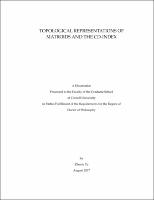## Topological Representations of Matroids and the cd-index##### Abstract
A fundamental achievement in the theory of matroids is the Topological Representation Theorem which says that every oriented matroid arises from an arrangement of pseudospheres. In 2003 Swartz extended this result to arbitrary matroids by using homotopy spheres. Later, Anderson and Engstrom also constructed topological representations of matroids by homotopy sphere arrangements. Inspired by Swartz's work, this thesis will show an explicit fully partitioned homotopy sphere $d$-arrangement $\mathcal{S}$ that is a CW-complex whose intersection lattice is the geometric lattice of the corresponding matroid for matroids of rank $\leq 4$. Moreover $\mathcal{S}$ has a $d$-sphere in it that is a regular CW-complex. This will allows us to look at how the flag $f$-vector formula of Billera, Ehrenborg and Readdy (BER) for oriented matroids applies to arbitrary matroids.
2017-08-30
Mathematics
##### Committee Chair
Swartz, Edward B.
##### Committee Member
Billera, Louis J.
Connelly, Robert
Mathematics
##### Degree Name
Ph. D., Mathematics
##### Degree Level
Doctor of Philosophy
##### Types
dissertation or thesis